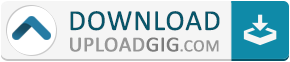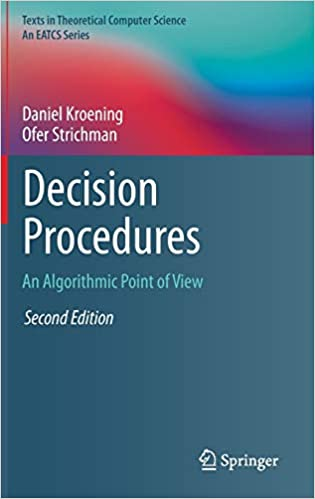#Do not remember me!

### Categories» » » Decision Procedures: An Algorithmic Point of View

## Decision Procedures: An Algorithmic Point of ViewEnglish | 377 pages | Springer; 2nd ed. 2016 edition (January 27, 2017) | 3662504960 | PDF | 3.72 Mb

A decision procedure is an algorithm that, given a decision problem, terminates with a correct yes/no answer. Here, the authors focus on theories that are expressive enough to model real problems, but are still decidable. Specifically, the book concentrates on decision procedures for first-order theories that are commonly used in automated verification and reasoning, theorem-proving, compiler optimization and operations research. The techniques described in the book draw from fields such as graph theory and logic, and are routinely used in industry. The authors introduce the basic terminology of satisfiability modulo theories and then, in separate chapters, study decision procedures for each of the following theories: propositional logic; equalities and uninterpreted functions; linear arithmetic; bit vectors; arrays; pointer logic; and quantified formulas.Name:* E-Mail: Security Code: *